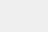# PyTorch 60 分钟入门教程：自动微分

1、TENSOR

`import torch`

```x = torch.ones(2, 2, requires_grad=True)
print(x)```

```tensor([[1., 1.],

```y = x + 2
print(y)```

```tensor([[3., 3.],
```

`print(y.grad_fn)`

```<AddBackward0 object at 0x7fe1db427470>
```

```z = y * y * 3
out = z.mean()

print(z, out)```

```tensor([[27., 27.],

`.requires_grad_( ... )` 会改变张量的 `requires_grad` 标记。输入的标记默认为  `False` ，如果没有提供相应的参数。

```a = torch.randn(2, 2)
a = ((a * 3) / (a - 1))
b = (a * a).sum()

```False
True
<SumBackward0 object at 0x7fe1db427dd8>```

`out.backward()`

`print(x.grad)`
输出：
```tensor([[4.5000, 4.5000],
[4.5000, 4.5000]])``````x = torch.randn(3, requires_grad=True)

y = x * 2
while y.data.norm() < 1000:
y = y * 2

print(y)```

`tensor([ -444.6791,   762.9810, -1690.0941], grad_fn=<MulBackward0>)`

```v = torch.tensor([0.1, 1.0, 0.0001], dtype=torch.float)
y.backward(v)

`tensor([1.0240e+02, 1.0240e+03, 1.0240e-01])`

```print(x.requires_grad)

```True
True
False```

`autograd` 和 `Function` 的文档在： https://pytorch.org/docs/autograd